# RS Aggarwal Solutions for Class 6 Maths Chapter 2 Factors and Multiples Exercise 2B

RS Aggarwal Solutions for Class 6 Maths, Chapter 2, Factors and Multiples Exercise 2B are given here. The second exercise of Chapter 2 has questions about the concept of divisibility tests, general properties of divisibility and steps which are followed to find prime numbers. RS Aggarwal Solutions can be used by students to solve problems without any error.

BYJU’S experts prepare solutions to help the students to gain a better academic score in a subject like Mathematics.

## Download PDF of RS Aggarwal Solutions for Class 6 Maths Chapter 2 Factors and Multiples Exercise 2B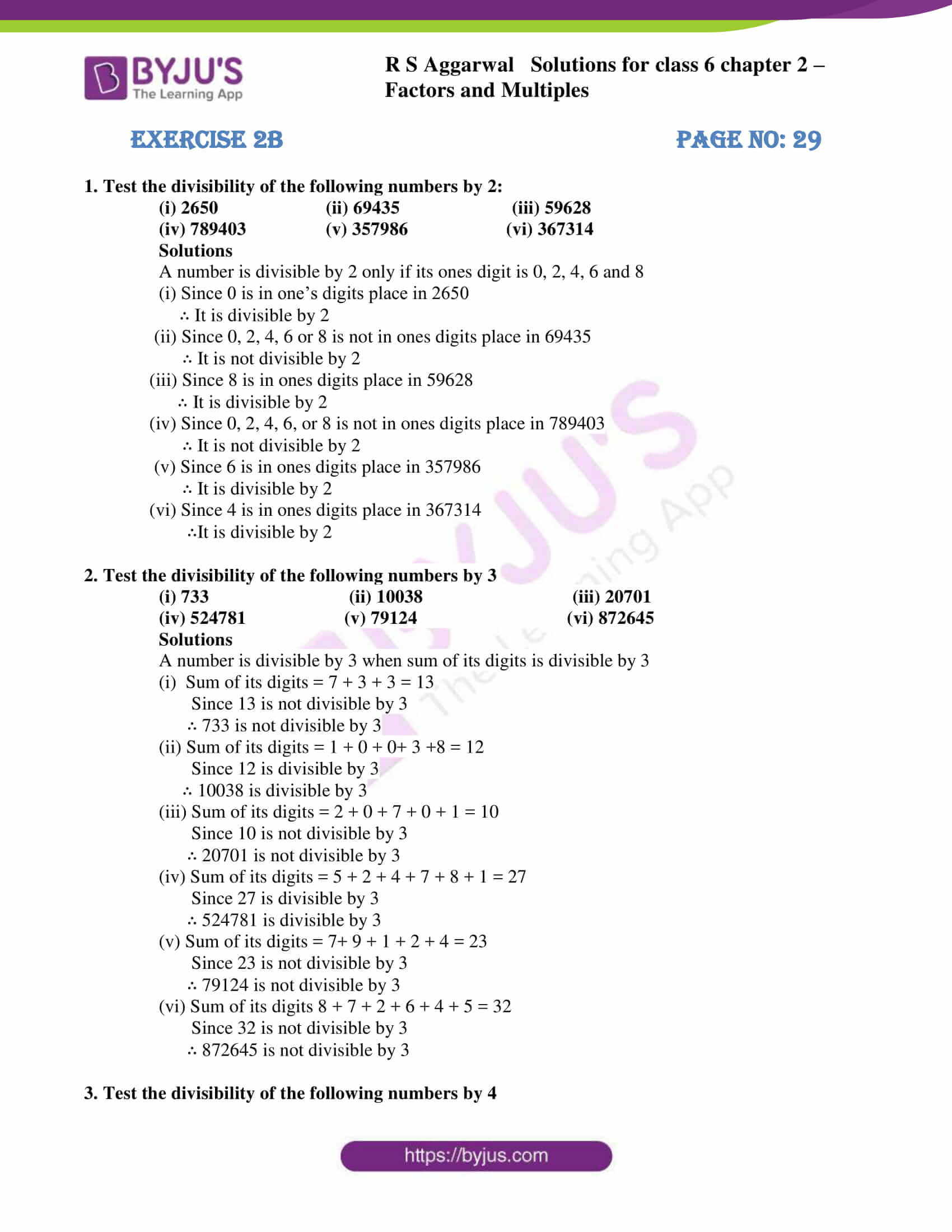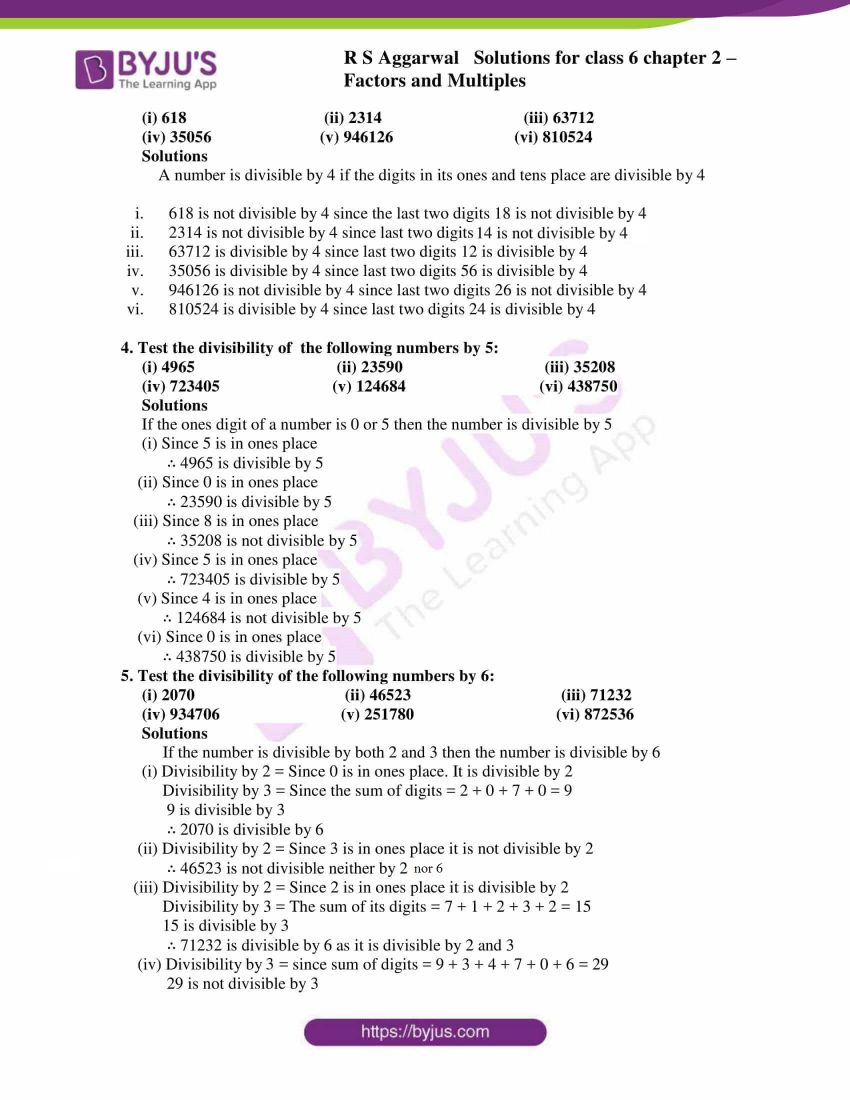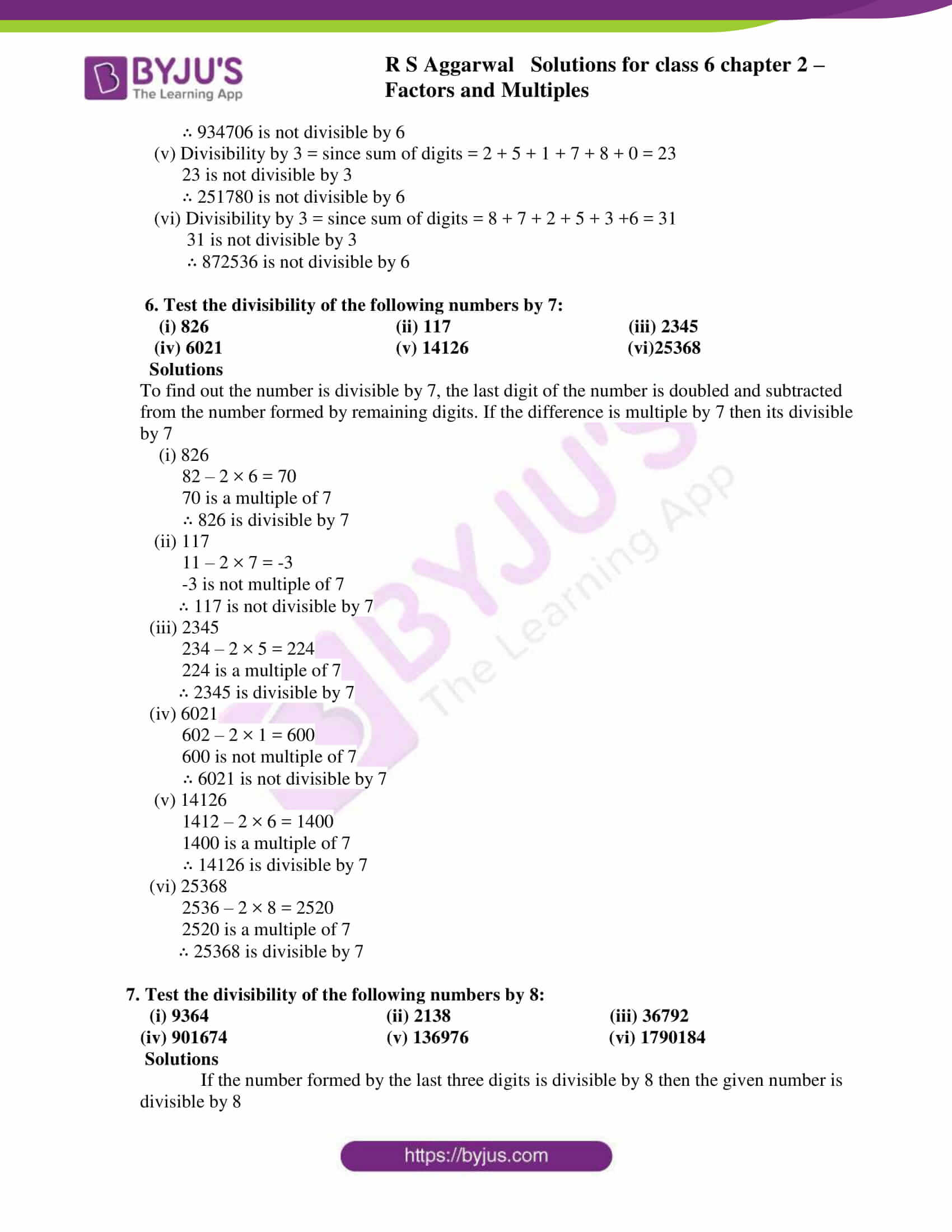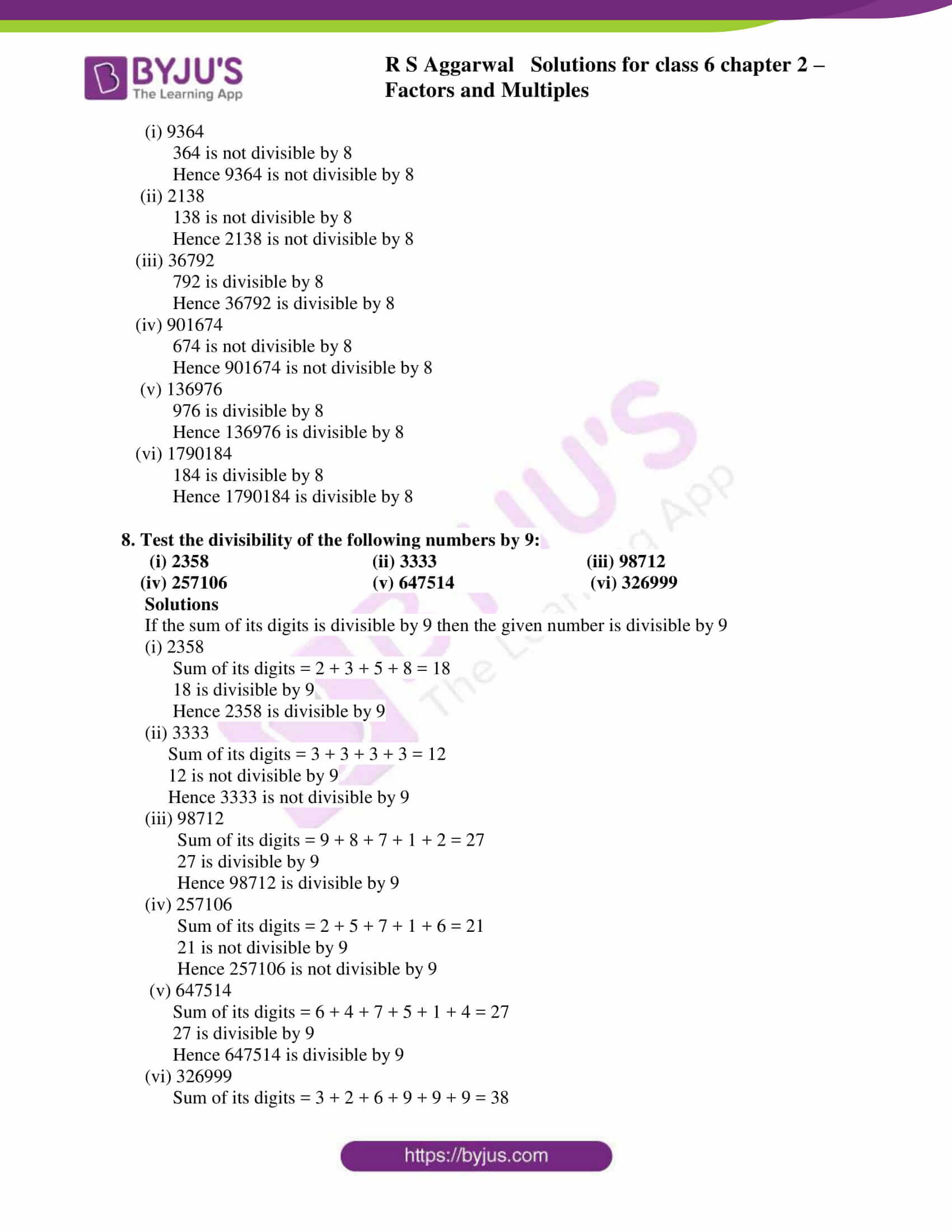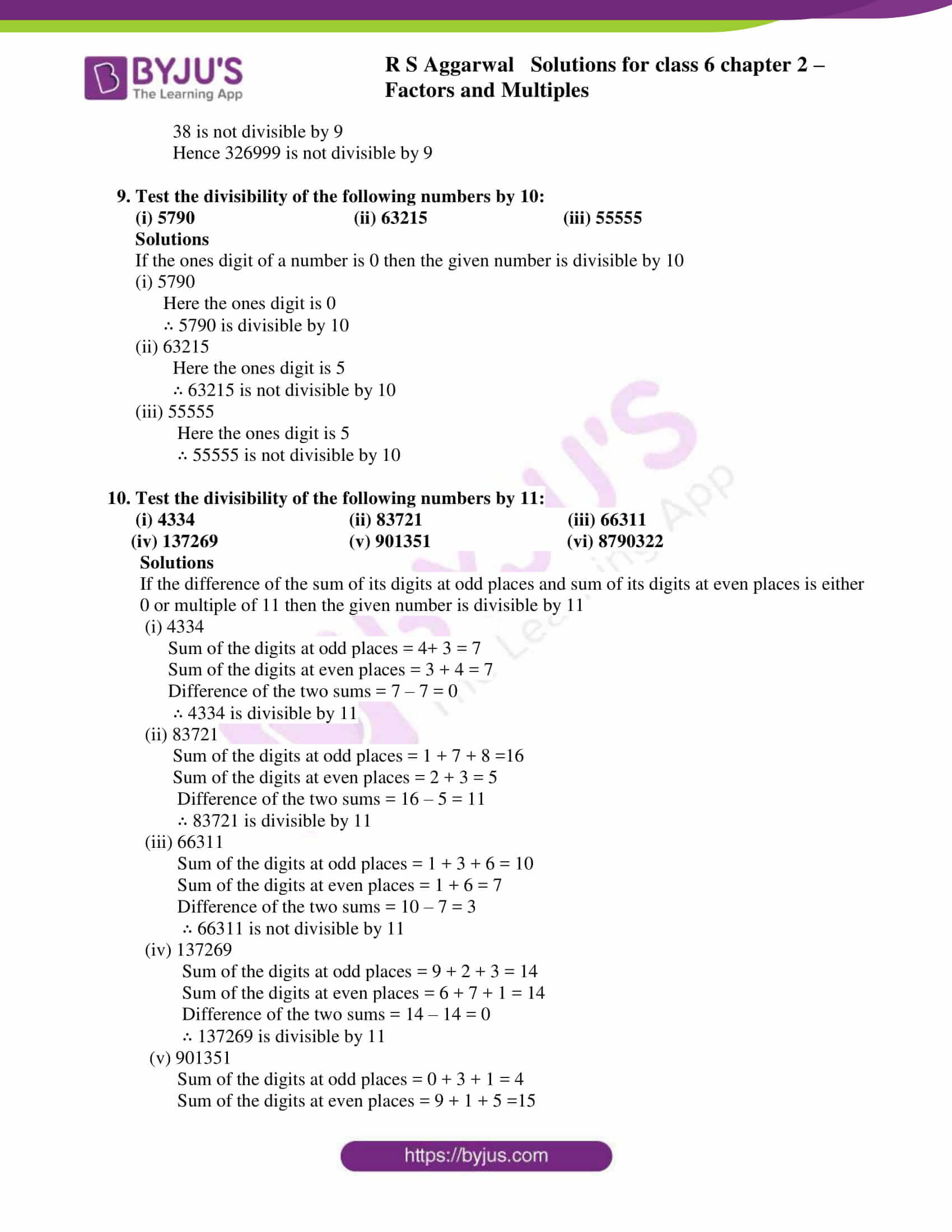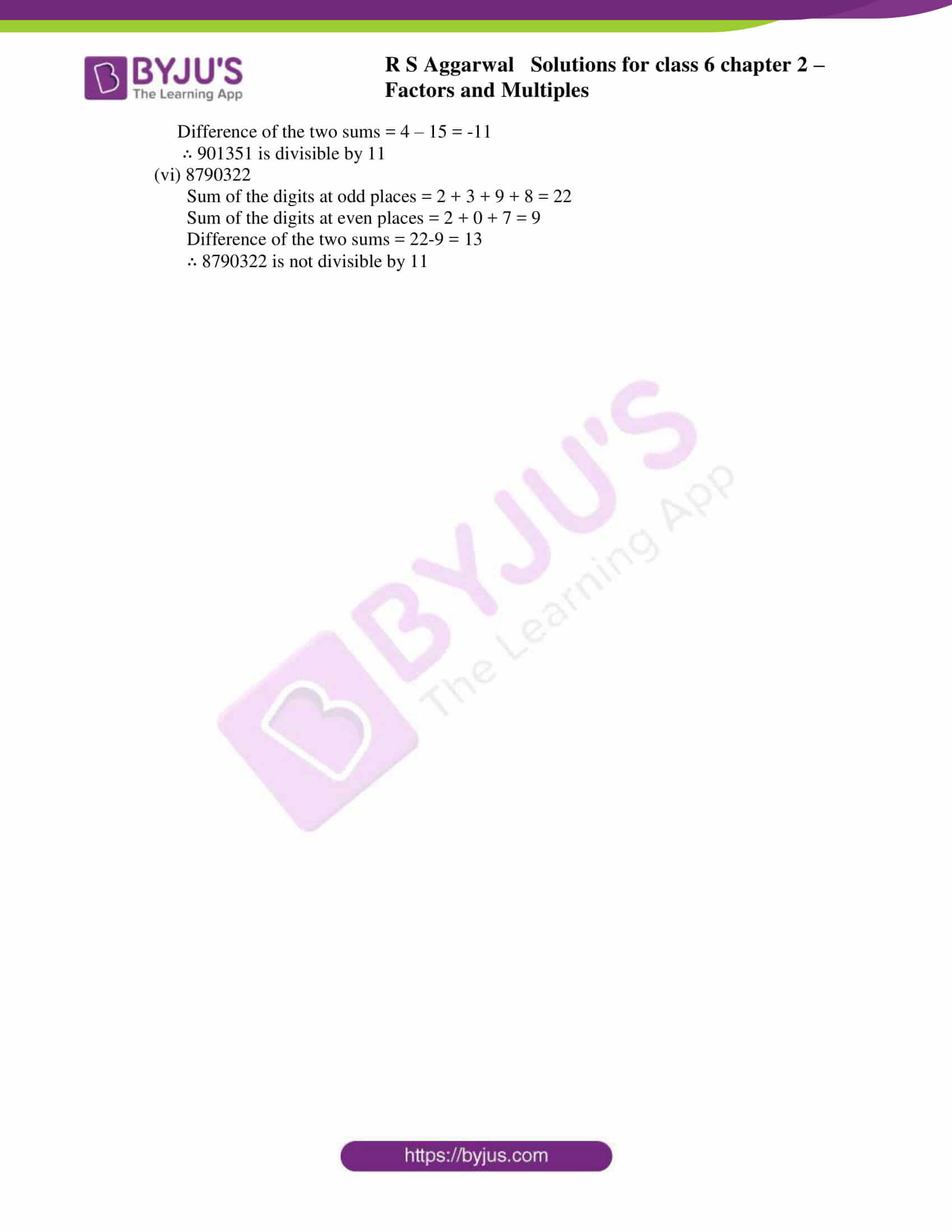### Access answers to Maths RS Aggarwal Solutions for Class 6 Chapter 2 Factors and Multiples Exercise 2B

1. Test the divisibility of the following numbers by 2:

(i) 2650 (ii) 69435 (iii) 59628

(iv) 789403 (v) 357986 (vi) 367314

Solutions

A number is divisible by 2 only if its ones digit is 0, 2, 4, 6 and 8

(i) Since 0 is in one’s digits place in 2650

∴ It is divisible by 2

(ii) Since 0, 2, 4, 6 or 8 is not in ones digits place in 69435

∴ It is not divisible by 2

(iii) Since 8 is in ones digits place in 59628

∴ It is divisible by 2

(iv) Since 0, 2, 4, 6, or 8 is not in ones digits place in 789403

∴ It is not divisible by 2

(v) Since 6 is in ones digits place in 357986

∴ It is divisible by 2

(vi) Since 4 is in ones digits place in 367314

∴It is divisible by 2

2. Test the divisibility of the following numbers by 3

(i) 733 (ii) 10038 (iii) 20701

(iv) 524781 (v) 79124 (vi) 872645

Solutions

A number is divisible by 3 when sum of its digits is divisible by 3

(i) Sum of its digits = 7 + 3 + 3 = 13

Since 13 is not divisible by 3

∴ 733 is not divisible by 3

(ii) Sum of its digits = 1 + 0 + 0+ 3 +8 = 12

Since 12 is divisible by 3

∴ 10038 is divisible by 3

(iii) Sum of its digits = 2 + 0 + 7 + 0 + 1 = 10

Since 10 is not divisible by 3

∴ 20701 is not divisible by 3

(iv) Sum of its digits = 5 + 2 + 4 + 7 + 8 + 1 = 27

Since 27 is divisible by 3

∴ 524781 is divisible by 3

(v) Sum of its digits = 7+ 9 + 1 + 2 + 4 = 23

Since 23 is not divisible by 3

∴ 79124 is not divisible by 3

(vi) Sum of its digits 8 + 7 + 2 + 6 + 4 + 5 = 32

Since 32 is not divisible by 3

∴ 872645 is not divisible by 3

3. Test the divisibility of the following numbers by 4

(i) 618 (ii) 2314 (iii) 63712

(iv) 35056 (v) 946126 (vi) 810524

Solutions

A number is divisible by 4 if the digits in its ones and tens place are divisible by 4

(i) 618 is not divisible by 4 since the last two digits 18 is not divisible by 4

(ii) 2314 is not divisible by 4 since last two digits 14 is not divisible by 4

(iii) 63712 is divisible by 4 since last two digits 12 is divisible by 4

(iv) 35056 is divisible by 4 since last two digits 56 is divisible by 4

(v) 946126 is not divisible by 4 since last two digits 26 is not divisible by 4

(vi) 810524 is divisible by 4 since last two digits 24 is divisible by 4

4. Test the divisibility of the following numbers by 5:

(i) 4965 (ii) 23590 (iii) 35208

(iv) 723405 (v) 124684 (vi) 438750

Solutions

If the ones digit of a number is 0 or 5 then the number is divisible by 5

(i) Since 5 is in ones place

∴ 4965 is divisible by 5

(ii) Since 0 is in ones place

∴ 23590 is divisible by 5

(iii) Since 8 is in ones place

∴ 35208 is not divisible by 5

(iv) Since 5 is in ones place

∴ 723405 is divisible by 5

(v) Since 4 is in ones place

∴ 124684 is not divisible by 5

(vi) Since 0 is in ones place

∴ 438750 is divisible by 5

5. Test the divisibility of the following numbers by 6:

(i) 2070 (ii) 46523 (iii) 71232

(iv) 934706 (v) 251780 (vi) 872536

Solutions

If the number is divisible by both 2 and 3 then the number is divisible by 6

(i) Divisibility by 2 = Since 0 is in ones place. It is divisible by 2

Divisibility by 3 = Since the sum of digits = 2 + 0 + 7 + 0 = 9

9 is divisible by 3

∴ 2070 is divisible by 6

(ii) Divisibility by 2 = Since 3 is in ones place it is not divisible by 2

∴ 46523 is not divisible neither by 2 nor 6

(iii) Divisibility by 2 = Since 2 is in ones place it is divisible by 2

Divisibility by 3 = The sum of its digits = 7 + 1 + 2 + 3 + 2 = 15

15 is divisible by 3

∴ 71232 is divisible by 6 as it is divisible by 2 and 3

(iv) Divisibility by 3 = since sum of digits = 9 + 3 + 4 + 7 + 0 + 6 = 29

29 is not divisible by 3

∴ 934706 is not divisible by 6

(v) Divisibility by 3 = since sum of digits = 2 + 5 + 1 + 7 + 8 + 0 = 23

23 is not divisible by 3

∴ 251780 is not divisible by 6

(vi) Divisibility by 3 = since sum of digits = 8 + 7 + 2 + 5 + 3 +6 = 31

31 is not divisible by 3

∴ 872536 is not divisible by 6

6. Test the divisibility of the following numbers by 7:

(i) 826 (ii) 117 (iii) 2345

(iv) 6021 (v) 14126 (vi)25368

Solutions

To find out the number is divisible by 7, the last digit of the number is doubled and subtracted from the number formed by remaining digits. If the difference is multiple by 7 then its divisible by 7

(i) 826

82 – 2 × 6 = 70

70 is a multiple of 7

∴ 826 is divisible by 7

(ii) 117

11 – 2 × 7 = -3

-3 is not multiple of 7

∴ 117 is not divisible by 7

(iii) 2345

234 – 2 × 5 = 224

224 is a multiple of 7

∴ 2345 is divisible by 7

(iv) 6021

602 – 2 × 1 = 600

600 is not multiple of 7

∴ 6021 is not divisible by 7

(v) 14126

1412 – 2 × 6 = 1400

1400 is a multiple of 7

∴ 14126 is divisible by 7

(vi) 25368

2536 – 2 × 8 = 2520

2520 is a multiple of 7

∴ 25368 is divisible by 7

7. Test the divisibility of the following numbers by 8:

(i) 9364 (ii) 2138 (iii) 36792

(iv) 901674 (v) 136976 (vi) 1790184

Solutions

If the number formed by the last three digits is divisible by 8 then the given number is divisible by 8

(i) 9364

364 is not divisible by 8

Hence 9364 is not divisible by 8

(ii) 2138

138 is not divisible by 8

Hence 2138 is not divisible by 8

(iii) 36792

792 is divisible by 8

Hence 36792 is divisible by 8

(iv) 901674

674 is not divisible by 8

Hence 901674 is not divisible by 8

(v) 136976

976 is divisible by 8

Hence 136976 is divisible by 8

(vi) 1790184

184 is divisible by 8

Hence 1790184 is divisible by 8

8. Test the divisibility of the following numbers by 9:

(i) 2358 (ii) 3333 (iii) 98712

(iv) 257106 (v) 647514 (vi) 326999

Solutions

If the sum of its digits is divisible by 9 then the given number is divisible by 9

(i) 2358

Sum of its digits = 2 + 3 + 5 + 8 = 18

18 is divisible by 9

Hence 2358 is divisible by 9

(ii) 3333

Sum of its digits = 3 + 3 + 3 + 3 = 12

12 is not divisible by 9

Hence 3333 is not divisible by 9

(iii) 98712

Sum of its digits = 9 + 8 + 7 + 1 + 2 = 27

27 is divisible by 9

Hence 98712 is divisible by 9

(iv) 257106

Sum of its digits = 2 + 5 + 7 + 1 + 6 = 21

21 is not divisible by 9

Hence 257106 is not divisible by 9

(v) 647514

Sum of its digits = 6 + 4 + 7 + 5 + 1 + 4 = 27

27 is divisible by 9

Hence 647514 is divisible by 9

(vi) 326999

Sum of its digits = 3 + 2 + 6 + 9 + 9 + 9 = 38

38 is not divisible by 9

Hence 326999 is not divisible by 9

9. Test the divisibility of the following numbers by 10:

(i) 5790 (ii) 63215 (iii) 55555

Solutions

If the ones digit of a number is 0 then the given number is divisible by 10

(i) 5790

Here the ones digit is 0

∴ 5790 is divisible by 10

(ii) 63215

Here the ones digit is 5

∴ 63215 is not divisible by 10

(iii) 55555

Here the ones digit is 5

∴ 55555 is not divisible by 10

10. Test the divisibility of the following numbers by 11:

(i) 4334 (ii) 83721 (iii) 66311

(iv) 137269 (v) 901351 (vi) 8790322

Solutions

If the difference of the sum of its digits at odd places and sum of its digits at even places is either 0 or multiple of 11 then the given number is divisible by 11

(i) 4334

Sum of the digits at odd places = 4+ 3 = 7

Sum of the digits at even places = 3 + 4 = 7

Difference of the two sums = 7 – 7 = 0

∴ 4334 is divisible by 11

(ii) 83721

Sum of the digits at odd places = 1 + 7 + 8 =16

Sum of the digits at even places = 2 + 3 = 5

Difference of the two sums = 16 – 5 = 11

∴ 83721 is divisible by 11

(iii) 66311

Sum of the digits at odd places = 1 + 3 + 6 = 10

Sum of the digits at even places = 1 + 6 = 7

Difference of the two sums = 10 – 7 = 3

∴ 66311 is not divisible by 11

(iv) 137269

Sum of the digits at odd places = 9 + 2 + 3 = 14

Sum of the digits at even places = 6 + 7 + 1 = 14

Difference of the two sums = 14 – 14 = 0

∴ 137269 is divisible by 11

(v) 901351

Sum of the digits at odd places = 0 + 3 + 1 = 4

Sum of the digits at even places = 9 + 1 + 5 =15

Difference of the two sums = 4 – 15 = -11

∴ 901351 is divisible by 11

(vi) 8790322

Sum of the digits at odd places = 2 + 3 + 9 + 8 = 22

Sum of the digits at even places = 2 + 0 + 7 = 9

Difference of the two sums = 22-9 = 13

∴ 8790322 is not divisible by 11

## RS Aggarwal Solutions for Class 6 Maths Chapter 2 Factors and Multiples Exercise 2B

Exercise 2B of RS Aggarwal Solutions for Class 6 Maths Chapter 2 Factors and Multiples is based on divisibility tests, general properties of divisibility and steps followed in finding prime numbers between the given set of numbers. Topics mentioned in other exercises are mentioned below:

• Finding prime and composite numbers from 1 to 100
• Prime factorization
• To find HCF
• Properties of HCF and LCM of given numbers

Students can learn the chapter clearly with the help of RS Aggarwal Solutions for Class 6, since the solutions are available in step by step manner. By practicing textbook problems, the students boost their self confidence while appearing for the exam.